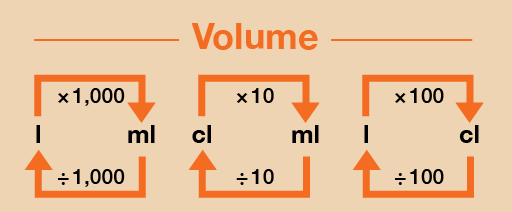Everyday maths 1 (Wales)

Start this free course now. Just create an account and sign in. Enrol and complete the course for a free statement of participation or digital badge if available.

Free course

# 4.2 Converting metric units of capacity

You will sometimes need to change between millilitres and litres. There are 1 000 millilitres in a litre.

Take a look at this metric conversion chart to refer to when you are carrying out the activity below.Figure 33 A conversion chart for volume

As mentioned earlier, capacity/volume can be measured in centilitres (cl), but it is more common to use millilitres (ml) and litres (l), so we will focus on converting between these here.

## Example: Converting units of capacity

1. Convert the following from litres into millilitres:
• a.7 litres = ? ml
• b.8.5 litres = ? ml
2. Convert the following from millilitres into litres:
• a.6 000 ml = ? litres
• b.2 750 ml = ? litres

### Method

1. As you can see from Figure 33, to convert from litres (l) to millilitres (ml), you need to multiply by 1 000:
• a.7 l × 1 000 = 7 000 ml
• b.8.5 l × 1 000 = 8 500 ml
2. If you want to convert from millilitres (ml) to litres (l) then you need to divide by 1 000:
• a.6 000 ml ÷ 1 000 = 6 l
• b.2 750 ml ÷ 1 000 = 2.75 l

Now try the following activity.

## Activity 16: Converting metric units of capacity

Calculate the following without using a calculator. You may wish to look back at Session 1 to remind you how to multiply [Tip: hold Ctrl and click a link to open it in a new tab. (Hide tip)] and divide by 1 000. Remember to check your answers.

1. What are the following measurements in litres?
• a.4 000 ml
• b.3 500 ml
• c.650 ml
• d.8 575 ml
2. What are the following measurements in millilitres?
• a.9 litres
• b.2.5 litres
• c.4.8 litres
• d.8.95 litres

### Answer

1. The answers are as follows:
• a.4 000 ml ÷ 1 000 = 4 litres
• b.3 500 ml ÷ 1 000 = 3.5 litres
• c.650 ml ÷ 1 000 = 0.65 litres
• d.8 575 ml ÷ 1 000 = 8.575 litres
2. The answers are as follows:
• a.9 litres × 1 000 = 9 000 ml
• b.2.5 litres × 1 000 = 2 500 ml
• c.4.8 litres × 1 000 = 4 800 ml
• d.8.95 litres × 1 000 = 8 950 ml
FSM_1_CYMRU

### Take your learning further

Making the decision to study can be a big step, which is why you'll want a trusted University. The Open University has 50 years’ experience delivering flexible learning and 170,000 students are studying with us right now. Take a look at all Open University courses.

If you are new to University-level study, we offer two introductory routes to our qualifications. You could either choose to start with an Access module, or a module which allows you to count your previous learning towards an Open University qualification. Read our guide on Where to take your learning next for more information.

Not ready for formal University study? Then browse over 1000 free courses on OpenLearn and sign up to our newsletter to hear about new free courses as they are released.

Every year, thousands of students decide to study with The Open University. With over 120 qualifications, we’ve got the right course for you.

Request an Open University prospectus371